# Genus of a curve

(diff) ← Older revision | Latest revision (diff) | Newer revision → (diff)

A numerical invariant of a one-dimensional algebraic variety defined over a field. The genus of a smooth complete algebraic curveis equal to the dimension of the space of regular differential-forms on(cf. Differential form). The genus of an algebraic curveis equal, by definition, to the genus of the complete algebraic curve birationally isomorphic to. For any integer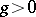there exists an algebraic curve of genus. An algebraic curve of genus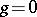over an algebraically closed field is a rational curve, i.e. it is birationally isomorphic to the projective line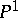. Curves of genus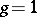(elliptic curves, cf. Elliptic curve) are birationally isomorphic to smooth cubic curves in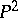. The algebraic curves of genus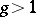fall into two classes: hyper-elliptic curves and non-hyper-elliptic curves. For non-hyper-elliptic curvesthe rational mappingdefined by the canonical class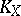of the complete smooth curve is an isomorphic imbedding. For a hyper-elliptic curvethe mappingis a two-sheeted covering of a rational curve,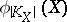, ramified atpoints.

Ifis a projective plane curve of degree, thenwhereis a non-negative integer measuring the deviation from smoothness of. Ifhas only ordinary double points, thenis equal to the number of singular points of. For a curvein space the following estimate is valid:whereis the degree ofin.

If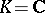is the field of complex numbers, then an algebraic curveis the same as a Riemann surface. In this case the smooth complex curveof genusis homeomorphic to the sphere withhandles.